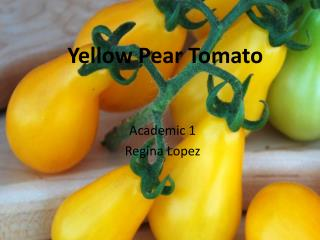DownloadDownload PresentationYellow Pear Tomato

# Yellow Pear Tomato

Télécharger la présentation## Yellow Pear Tomato

- - - - - - - - - - - - - - - - - - - - - - - - - - - E N D - - - - - - - - - - - - - - - - - - - - - - - - - - -
##### Presentation Transcript

1. Yellow Pear Tomato Academic 1 Regina Lopez

2. Small bright yellow bite-sized fruit. Flavor is delicious. • Seasonality: Mid Season  • Fruit Weight: 4 ounces • Days to Maturity: 75 days • Sun: Full Sun  • Spread: 18 inches • 1/4 inch deep • (60-70°F). • 20-30 inches apart • 3-4 feet between rows • Days to emergence: 6-14

3. Calculations • To find my growth rate, I had to first find the. I divided the maximum height of the plant, 18 inches, and divided that by the maturity growth 75 days after dividing I got my slope and that was 0.24. Then to find how much the plant will go until the maturity growth rate we had to multiply each day by 0.24 to find out how much it will grow each day. Then after that we had to make a graph showing the growth rate. That was all we had to find after that. How could this be used in real life? I think it can be used in real life by doing the exact same steps we used to find the height and growth rates and equations and you’ll still get the results you need for whatever you’re figuring out. Can this be used for other real life things? No, I don’t think it can be used in real life activities because it is used for finding growth rates or plants. • Growth rate= max height/max days y=mx+b y= 2in/78days= 0.24

4. Ways it can be used in real life • The calculations can be used in real life situations by helping out other farmers figure out how they can plant and grow there plants, they need to figure out how many days, how long it will take to grow it, how much space is needed to grow it. This can be used I think in pretty much any real life situations to figure out for example like the rate of a heart beat, how fast something can be, how long something could be.

5. Bibliography • http://www.territorialseed.com/product/1153/403 • http://rareseeds.com/yellow-pear.html

6. Graph Two (75,18) 18=a(75-0)²+0 18=a(75)² 18=a5625 18/5625 0.0032=a Y=ax² Y=0.0032(x)²

7. How tall will it be after 60 days? Y=.032x² Y=.032(60)² Y=.032(3600) Y=115.2 How many days until the plant is 4” high? 4=.032x² 4/.032=x²=125=x² 11.2=x How tall will the plant be after 30 days? Y=.032x² Y=.032(30)² Y=.032(900) Y=28.8 How many days until the plant is 8” high? 8=.032x² 250=x² 15.8=x

8. Comparison The difference between the two graphs would be that the first graph was going straight up and the second graph curves. The first graph wasn’t that realistic but then the second graph is realistic because the plants grow in a curve. Sometimes they don’t grow in straight. The plants grow in a curve way because no plants grows directly straight. The weather also helps the plant grow because when it rains is give the plant water to drink and feeds the seed so that it grows everyday.

9. Real life • The growth rate of a baby • Growth of humans & animals • Plants • Greenhouse plants • Growth of hair • Height of people • Basically anything that can grow exponentially.